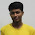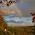## Tuesday, January 10, 2012

### a2 + b2 = c2I was going through the problems posted in Project Euler and was suddenly struck with this problem Pythagorean Triplet

Find the only Pythagorean triplet, {a, b, c}, for which a + b + c = 1000I immediately Googled on how to find Pythagorean triplet and here it goes
Given any two arbitrary numbers ‘m’ and ‘n’
Take a = m2 - n2
b = 2mn
c = m2 +n2
Hence a2+b2 = c2 and we get a tripletwell this method gives an idea how to form new triplets but not to find a triplet containing a given numberI was really interested in thisnow consider the expansion (x + a)2 = x2+ a2 +2ax
if a2 + 2ax = some square number .say y2 then we get a triplet ( x, y, x+a )
so for a given 'y' we have to find 'x' and 'a' to get the triplet
( x, y, x+a )
y2 = a2 + 2ax = a ( a + 2x )
a2 = y2 – 2ax
Clearly ‘a’ should be lesser than square root o
f ‘y’

Steps :
1. Divide y2 by 'a'
2. Subtract 'a' from it
3. You shd get an EVEN number else choose another 'a' and goto 1
For eg. take 36… Factors are – 1 2 3 4 6 9 12 18 36
so ‘a’ can take value { 1 2 3 4 }

substituting a = 1 or 3 or 4 we get fractional values of ‘x’ hence we omit them

hence taking a=2 we get x=8
hence the triplet is (8, 6, 8+2) i.e. ( 8, 6, 10)hence we can generate any triplet for a given number by choosing proper value for 'a'

Furthur observations :1) 'a' is odd for a given odd square number and vice versa
2) Special case : consider y2= 4 or 1 . there are no values for 'a' in this case as square root are 2 and 1. Hence we cannot have a triplet containing 1 or 2

3) The above statement also proves that "There cannot be a right angled triangle with integer sides and any one of sides equal to 1 or 2"

I Googled on this topic and found nothing regarding thisto be continued1.2.3.4.